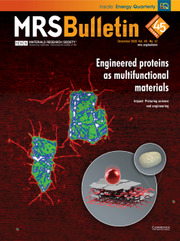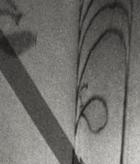Home
Hostname: page-component-544b6db54f-bkjnw Total loading time: 0.415 Render date: 2021-10-25T05:28:09.649Z Has data issue: true Feature Flags: { "shouldUseShareProductTool": true, "shouldUseHypothesis": true, "isUnsiloEnabled": true, "metricsAbstractViews": false, "figures": true, "newCiteModal": false, "newCitedByModal": true, "newEcommerce": true, "newUsageEvents": true }MRS Bulletin

# Metastability alloy design

Published online by Cambridge University Press:  09 April 2019

## AbstractThis article reviews the concept of metastability in alloy design. While most materials are thermodynamically metastable at some stage during synthesis and service, we discuss here cases where metastable phases are not coincidentally inherited from processing, but rather are engineered. Specifically, we aim at compositional (partitioning), thermal (kinetics), and microstructure (size effects and confinement) tuning of metastable phases so that they can trigger athermal transformation effects when mechanically, thermally, or electromagnetically loaded. Such a concept works both at the bulk scale and also at a spatially confined microstructure scale, such as at lattice defects. In the latter case, local stability tuning works primarily through elemental partitioning to dislocation cores, stacking faults, interfaces, and precipitates. Depending on stability, spatial confinement, misfit, and dispersion, both bulk and local load-driven athermal transformations can equip alloys with substantial gain in strength, ductility, and damage tolerance. Examples include self-organized metastable nanolaminates, austenite reversion steels, metastable medium- and high-entropy alloys, as well as steels and titanium alloys with martensitic phase transformation and twinning-induced plasticity effects.

## Keywords

Type
Computational Design And Development Of Alloys
Information
MRS Bulletin , April 2019 , pp. 266 - 272

## Access options

Get access to the full version of this content by using one of the access options below. (Log in options will check for institutional or personal access. Content may require purchase if you do not have access.)

## References

Raabe, D., Sandlöbes, S., Millán, J., Ponge, D., Assadi, H., Herbig, M., Choi, P.-P., Acta Mater. 61, 6132 (2013).CrossRefGoogle Scholar
Raabe, D., Herbig, M., Sandlöbes, S., Li, Y., Tytko, D., Kuzmina, M., Choi, P.-P., Curr. Opin. Solid State Mater. Sci. 18, 253 (2014).CrossRefGoogle Scholar
Kuzmina, M., Ponge, D., Raabe, D., Acta Mater. 86, 182 (2015).CrossRefGoogle Scholar
Makineni, S.K., Lenz, M., Kontis, P., Li, Z., Kumar, A., Felfer, P.J., Neumeier, S., Herbig, M., Spiecker, E., Raabe, D., Gault, B., JOM 70, 1736 (2018).CrossRefGoogle Scholar
Li, Y., Raabe, D., Herbig, M., Choi, P.-P., Goto, S., Kostka, A., Yarita, H., Borchers, C., Kirchheim, R., Phys. Rev. Lett. 113, 106104 (2014).CrossRefGoogle Scholar
Grässel, O., Krüger, L., Frommeyer, G., Meyer, L.W., Int. J. Plast. 16, 1391 (2000).CrossRefGoogle Scholar
Zaefferer, S., Ohlert, J., Bleck, W., Acta Mater. 52, 2765 (2004).CrossRefGoogle Scholar
Liu, J., Chen, C., Feng, Q., Fang, X., Wang, H., Liu, F., Lu, J., Raabe, D., Mater. Sci. Eng. A 703, 236 (2017).CrossRefGoogle Scholar
Raabe, D., Acta Mater. 45, 1137 (1997).CrossRefGoogle Scholar
Frommeyer, G., Brüx, U., Neumann, P., ISIJ Int . 43, 438 (2003).CrossRefGoogle Scholar
Han, J., da Silva, A.K., Ponge, D., Raabe, D., Lee, S.-M., Lee, Y.-K., Lee, S.-I., Hwang, B., Acta Mater. 122, 199 (2017).CrossRefGoogle Scholar
Allain, S., Chateau, J.P., Bouaziz, O., Steel Res. Int. 73, 299 (2002).CrossRefGoogle Scholar
Bouaziz, O., Allain, S., Scott, C.P., Cugy, P., Barbier, D., Curr. Opin. Solid State Mater. Sci. 15, 141 (2011).CrossRefGoogle Scholar
Saeed-Akbari, A., Mosecker, L., Schwedt, A., Bleck, W., Metall. Mater. Trans. A 43, 1688 (2012).CrossRefGoogle Scholar
Gutierrez-Urrutia, I., Raabe, D., Acta Mater. 59, 6449 (2011).CrossRefGoogle Scholar
Steinmetz, D.R., Japel, T., Wietbrock, B., Eisenlohr, P., Gutierrez-Urrutia, I., Saeed-Akbari, A., Hickel, T., Roters, F., Raabe, D., Acta Mater. 61, 494 (2013).CrossRefGoogle Scholar
Idrissi, H., Renard, K., Schryvers, D., Jacques, P.J., Scr. Mater. 63, 961 (2010).CrossRefGoogle Scholar
Gutierrez-Urrutia, I., Zaefferer, S., Raabe, D., Mater. Sci. Eng. A 527, 3552 (2010).CrossRefGoogle Scholar
Choi, W.S., Sandlöbes, S., Malyar, N.V., Kirchlechner, C., Korte-Kerzel, S., Dehm, G., De Cooman, B.C., Raabe, D., Acta Mater. 132, 162 (2017).CrossRefGoogle Scholar
Herrera, C., Ponge, D., Raabe, D., Acta Mater. 59, 4653 (2011).CrossRefGoogle Scholar
Edmonds, D.V., He, K., Rizzo, F.C., De Cooman, B.C., Matlock, D.K., Speer, J.G., Mater. Sci. Eng. A 438, 25 (2006).CrossRefGoogle Scholar
Speer, J.G., Matlock, D.K., De Cooman, B.C., Schroth, J.G., Acta Mater. 51, 2611 (2003).CrossRefGoogle Scholar
Toji, Y., Matsuda, H, Herbig, M., Choi, P.P., Raabe, D., Acta Mater. 65, 215 (2014).CrossRefGoogle Scholar
Toji, Y., Miyamoto, G., Raabe, D., Acta Mater. 86, 137 (2015).CrossRefGoogle Scholar
Li, Z., Tasan, C.C., Pradeep, K.G., Raabe, D., Acta Mater. 131, 323 (2017).CrossRefGoogle Scholar
Gludovatz, B., Hohenwarter, A., Catoor, D., Chang, E.H., George, E.P., Ritchie, R.O., Science 345, 1153 (2014).CrossRefGoogle Scholar
Yao, M.J., Pradeep, K.G., Tasan, C.C., Raabe, D., Scr. Mater. 72, 5 (2014).CrossRefGoogle Scholar
Deng, Y., Tasan, C.C., Pradeep, K.G., Springer, H., Kostka, A., Raabe, D., Acta Mater. 94, 124 (2015).CrossRefGoogle Scholar
Laplanche, G., Kostka, A., Horst, O., Eggeler, G., George, E., Acta Mater. 118, 152 (2016).CrossRefGoogle Scholar
Li, Z.M., Pradeep, K.G., Deng, Y., Raabe, D., Tasan, C.C., Nature 534, 227 (2016).CrossRefGoogle Scholar
He, F., Wang, Z., Wu, Q., Li, J., Wang, J., Liu, C.T., Scr. Mater. 126, 15 (2017).CrossRefGoogle Scholar
Li, Z.M., Raabe, D., JOM 69, 2099 (2017).CrossRefGoogle Scholar
Ma, D., Yao, M., Pradeep, K.G., Tasan, C.C., Springer, H., Raabe, D., Acta Mater. 98, 288 (2015).CrossRefGoogle Scholar
Jo, Y.H., Jung, S., Choi, W.M., Sohn, S.S., Kim, H.S., Lee, B.J., Kim, N.J., Lee, S., Nat. Commun. 8, 15719 (2017).CrossRefGoogle Scholar
Wu, Z., Bei, H., Pharr, G.M., George, E.P., Acta Mater. 81, 428 (2014).CrossRefGoogle Scholar
Huang, S., Li, W., Lu, S., Tian, F.Y., Shen, J., Holmström, E., Vitos, L., Scr. Mater. 108, 44 (2015).CrossRefGoogle Scholar
Huang, S., Huang, H., Li, W., Kim, D., Lu, S., Li, X., Holmström, E., Kwon, S.K., Vitos, L., Nat. Commun. 9, 2381 (2018).CrossRefGoogle Scholar
Zaddach, A.J., Niu, C., Koch, C.C., Irving, D.L., JOM 65, 1780 (2013).CrossRefGoogle Scholar
Liu, S.F., Wu, Y.D., Wang, H.T., He, J.Y., Liu, J.B., Chen, C.X., Liu, X.J., Wang, H., Lu, Z.P., Intermetallics 93, 269 (2017).CrossRefGoogle Scholar
Gludovatz, B., Hohenwarter, A., Thurston, K.V.S., Bei, H.B., Wu, Z.G., George, E.P., Ritchie, R.O., Nature Commun . 7, 10602 (2016).CrossRefGoogle Scholar
Wang, M.M., Li, Z.M., Raabe, D., Acta Mater. 147, 236 (2018).CrossRefGoogle Scholar
Slone, C.E., Chakraborty, S., Miao, J., George, E.P., Mills, M.J., Niezgoda, S.R., Acta Mater. 158, 38 (2018).CrossRefGoogle Scholar
Okamoto, N.L., Fujimoto, S., Kambara, Y., Kawamura, M., Chen, Z.M., Matsunoshita, H., Tanaka, K., Inui, H., George, E.P., Sci. Rep. 6, 35863 (2016).CrossRefGoogle Scholar
Raabe, D., Tasan, C.C., Springer, H., Bausch, M., Steel Res. Int. 86, 1127 (2015).CrossRefGoogle Scholar
Ma, L.L., Wang, L., Nie, Z.H., Wang, F.C., Xue, Y.F., Zhou, J.L., Cao, T.Q., Wang, Y.D., Yang, R., Acta Mater. 128, 12 (2017).CrossRefGoogle Scholar
Li, Z., Körmann, F., Grabowski, B., Neugebauer, J., Raabe, D., Acta Mater. 136, 262 (2017).CrossRefGoogle Scholar
Moon, J., Qi, Y., Tabachnikova, E., Estrin, Y., Choi, W.M., Joo, S.H., Lee, B.J., Podolskiy, A., Tikhonovsky, M., Kim, H.S., Mater. Lett. 202, 86 (2017).CrossRefGoogle Scholar
Koyama, M., Zhang, Z., Wang, M., Ponge, D., Raabe, D., Tsuzaki, K., Noguchi, H., Tasan, C.C., Science 355, 1055 (2017).CrossRefGoogle Scholar
Kuzmina, M., Herbig, M., Ponge, D., Sandlöbes, S., Raabe, D., Science 349, 1080 (2015).CrossRefGoogle Scholar
Kwiatkowski da Silva, A., Ponge, D., Peng, Z., Inden, G., Lu, Y., Breen, A., Gault, B., Raabe, D., Nature Commun . 9, 1137 (2018).CrossRefGoogle Scholar
Calcagnotto, M., Ponge, D., Demir, E., Raabe, D., Mater. Sci. Eng. A 527, 2738 (2010).CrossRefGoogle Scholar
Wang, M.M., Tasan, C.C., Ponge, D., Kostka, A., Raabe, D., Acta Mater. 79, 268 (2014).CrossRefGoogle Scholar
Wang, M.M., Tasan, C.C., Ponge, D., Dippel, A.C., Raabe, D., Acta Mater. 85, 216 (2015).CrossRefGoogle Scholar
Wang, M.M., Tasan, C.C., Ponge, D., Dippel, A.C., Raabe, D., Acta Mater. 111, 262 (2016).CrossRefGoogle Scholar
Fowler, R.H., Guggenheim, E.A., Statistical Thermodynamics (Macmillan, New York, 1939).Google Scholar
Kwiatkowski da Silva, A., Leyson, G., Kuzmina, M., Ponge, D., Herbig, M., Sandlöbes, S., Gault, B., Neugebauer, J., Raabe, D., Acta Mater. 124, 305 (2017).CrossRefGoogle Scholar
Zhang, J., Tasan, C.C., Lai, M.J., Dippel, A.-C., Raabe, D., Nat. Commun. 8, 14210 (2017).CrossRefGoogle Scholar
Lai, M.J., Li, T., Raabe, D., Acta Mater. 151, 67 (2018).CrossRefGoogle Scholar
Lai, M.J., Tasan, C.C., Raabe, D., Acta Mater. 111, 173 (2016).CrossRefGoogle Scholar
Lai, M.J., Tasan, C.C., Zhang, J., Grabowski, B., Huang, L.F., Raabe, D., Acta Mater. 92, 55 (2015).CrossRefGoogle Scholar
Lai, M.J., Tasan, C.C., Raabe, D., Acta Mater. 100, 290 (2015).CrossRefGoogle Scholar
Dumay, A., Chateau, J.P., Allain, S., Migot, S., Bouaziz, O., Mater. Sci. Eng. A 483, 184 (2008).CrossRefGoogle Scholar
Pisarik, S.T., Van Aken, D.C., Metall. Mater. Trans. A 47, 1009 (2016).CrossRefGoogle Scholar
Lai, M.J., Li, Y.J., Lillpopp, L, Ponge, D., Will, S., Raabe, D., Acta Mater. 155, 222 (2018).CrossRefGoogle Scholar
Pierce, D.T., Jimenez, J.A., Bentley, J., Raabe, D., Oskay, C., Wittig, J.E., Acta Mater. 68, 238 (2014).CrossRefGoogle Scholar
Springer, H., Raabe, D., Acta Mater. 60, 4950 (2012).CrossRefGoogle Scholar
Gutierrez-Urrutia, I., Raabe, D., Acta Mater. 60, 5791 (2012).CrossRefGoogle Scholar
Li, Z., Ludwig, A., Savan, A., Springer, H., Raabe, D., J. Mater. Res. 33, 3156 (2018).CrossRefGoogle Scholar
Luo, H., Li, Z., Raabe, D., Sci. Rep. 7, 9892 (2017).CrossRefGoogle Scholar
Luo, H., Lu, W., Fang, X., Ponge, D., Li, Z., Raabe, D., Mater. Today (2018), doi:10.1016/j.mattod.2018.07.015.Google Scholar
Mahajan, S., Green, M.L., Brasen, D., Metall. Trans. A. 8, 283 (1977).CrossRefGoogle Scholar
Christian, J.W., Mahajan, S., Prog. Mater. Sci. 39, 1 (1995).CrossRefGoogle Scholar
Mahato, B., Shee, S.K., Sahu, T., Chowdhury, S.G., Sahu, P., Porter, D.A., Acta Mater. 86, 69 (2015).CrossRefGoogle Scholar
Li, Z., Tasan, C.C., Springer, H., Gault, B., Raabe, D., Sci. Rep. 7, 40704 (2017).CrossRefGoogle Scholar
Senkov, O.N., Scott, J.M., Senkova, S.V., Miracle, D.B., J. Alloys Compd. 509, 6043 (2011).CrossRefGoogle Scholar
Senkov, O.N., Senkova, S.V., Miracle, D.B., Woodward, C., Mater. Sci. Eng. A 565, 51 (2013).CrossRefGoogle Scholar
Lei, Z., Liu, X., Wu, Y., Wang, H., Jiang, S., Wang, S., Hui, X., Wu, Y., Gault, B., Kontis, P., Raabe, D., Gu, L., Zhang, Q., Chen, H., Wang, H., Liu, J., An, K., Zeng, Q., Nieh, T.G., Lu, Z., Nature 563, 546 (2018).CrossRefGoogle Scholar
Springer, H., Belde, M., Raabe, D., Mater. Des. 90, 1100 (2016).CrossRefGoogle Scholar
Springer, H., Belde, M., Raabe, D., Mater. Sci. Eng. A 582, 235 (2013).CrossRefGoogle Scholar
Belde, M., Springer, H., Inden, G., Raabe, D., Acta Mater. 86, 1 (2015).CrossRefGoogle Scholar

# Send article to Kindle

Note you can select to send to either the @free.kindle.com or @kindle.com variations. ‘@free.kindle.com’ emails are free but can only be sent to your device when it is connected to wi-fi. ‘@kindle.com’ emails can be delivered even when you are not connected to wi-fi, but note that service fees apply.

Find out more about the Kindle Personal Document Service.

Metastability alloy design
Available formats
×

# Send article to Dropbox

To send this article to your Dropbox account, please select one or more formats and confirm that you agree to abide by our usage policies. If this is the first time you use this feature, you will be asked to authorise Cambridge Core to connect with your <service> account. Find out more about sending content to Dropbox.

Metastability alloy design
Available formats
×

# Send article to Google Drive

To send this article to your Google Drive account, please select one or more formats and confirm that you agree to abide by our usage policies. If this is the first time you use this feature, you will be asked to authorise Cambridge Core to connect with your <service> account. Find out more about sending content to Google Drive.

Metastability alloy design
Available formats
×
×